# Step 2: Custom Blocks with Function Templates - 2022.1 English

## Vitis Model Composer Tutorial (UG1498)

Document ID
UG1498
Release Date
2022-07-25
Version
2022.1 English
In this step we will walk through an example to do the following:
• To create a custom block that supports inputs of different sizes.
• To create a custom block that accepts signals with different fixed-point lengths and fractional lengths.
• To perform simple arithmetic operations using template variables.
1. Navigate to the HLS_Library/Lab2/Section2 folder and open design.slx.
2. Double-click the template_design.h file to view the source code in the MATLAB Editor. There are two functions: Demux and Mux. These two functions are a demultiplexing and multiplexing of inputs as shown in the following figure.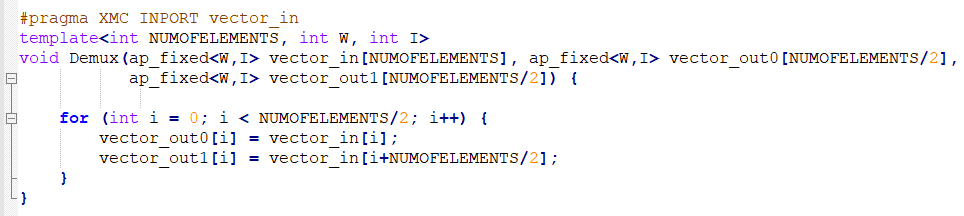3. In the piece of code, note the ```#pragma XMC INPORT vector_in```. This is a way to manually specify port directions using pragmas. Here, we are specifying the function argument `vector_in` as the input port. Similarly, we can define `XMC OUTPORT` also.
Note: For additional information about specifying ports, see Vitis Model Composer User Guide (UG1483).
4. Notice the use of template before the function declaration. To support the inputs of different sizes, `NUMOFELEMENTS` is declared as a parameter and used the same while defining an array `vector_in` as shown in the following figure. This allows you to connect signals of different sizes to the input port of the block.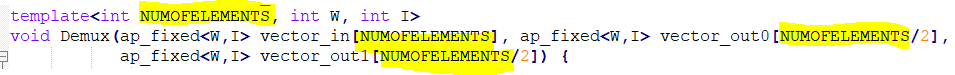5. Notice the template parameters `W` and `I` which are declared to accept signals with different word lengths and integer lengths.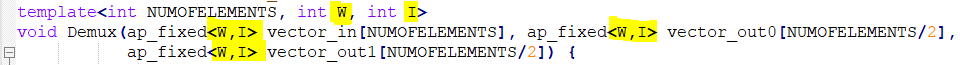6. Observe the arithmetic operations performed using template variables as shown below, indicating the output signal length is half of the input signal length.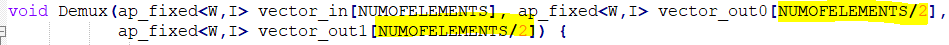7. Similar explanation follows for Mux function.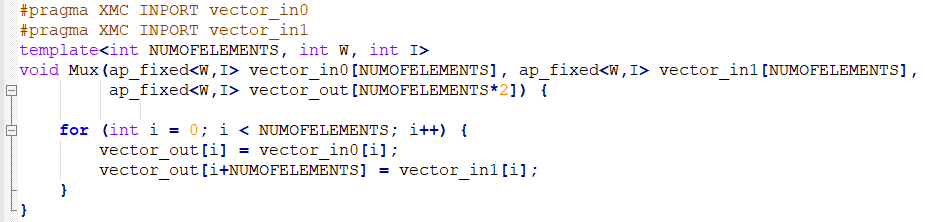Now create the library blocks for Mux and Demux functions using the `xmcImportFunction` command and complete the design below with custom blocks.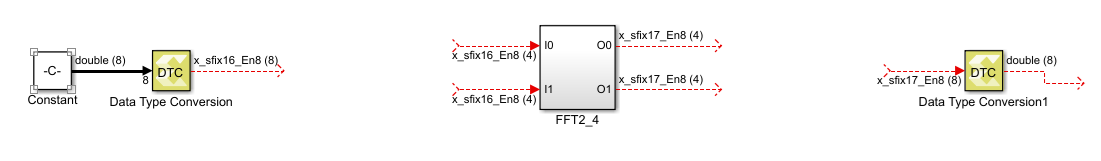8. Double-click the import_function.m script file in the MATLAB command window and observe the following commands that generate library blocks to embed into your actual design.
``````>>xmcImportFunction('design_lib',{'Demux'},'template_design.h',{},{},'override','unlock')
>>xmcImportFunction('design_lib',{'Mux'},'template_design.h',{},{},'override','unlock')
``````
Note: The same library is specified for both the functions.
9. Run the import_function.m script from the MATLAB command line:
``>>run('import_function.m')``
10. Observe the generated library blocks in the design_lib.slx library model file and save it to working directory.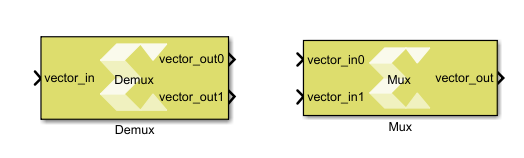11. Copy the Demux and Mux blocks and paste them in the design.slx file and connect them as shown in the following figure.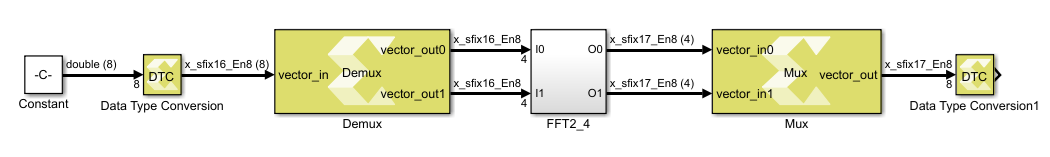12. Note the following after embedding the custom blocks:
1. Double-click the Constant block and observe the vector input of type double. SSR is a workspace variable, initially set to 8 from the initFcn model callback.
2. Using the Data Type Conversion (DTC) block, double type is converted to fixed type with 16-bit word length and 8-bit fractional length.

Input is configurable to any word length since the design is templatized.

3. Double-click the Demux block and observe the Template parameters section and Dimension column in the Interface section of the function tab.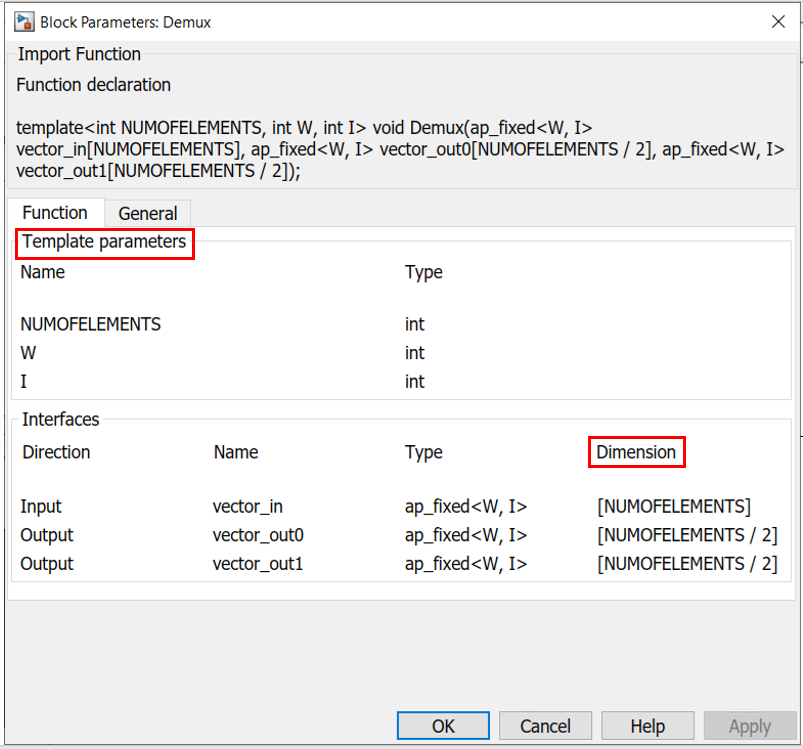4. Next, double-click the Mux block and observe the Template parameters and Dimension.
13. Add a Display block at the input and output as shown in the following figure and simulate the model to observe the results.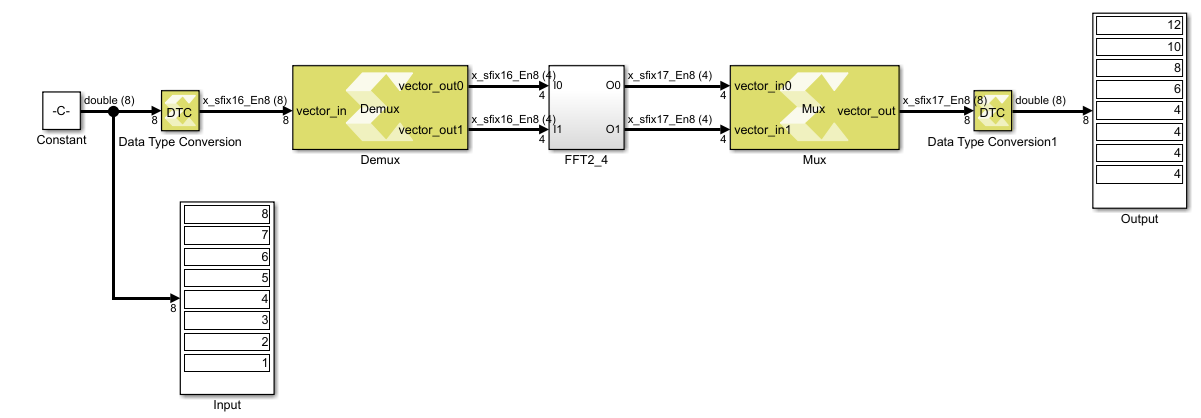1. Double-click the DTC block.
2. In the Block Parameters dialog box, change the Word length from 16 to 32.
3. Change the Fractional length from 8 to 16.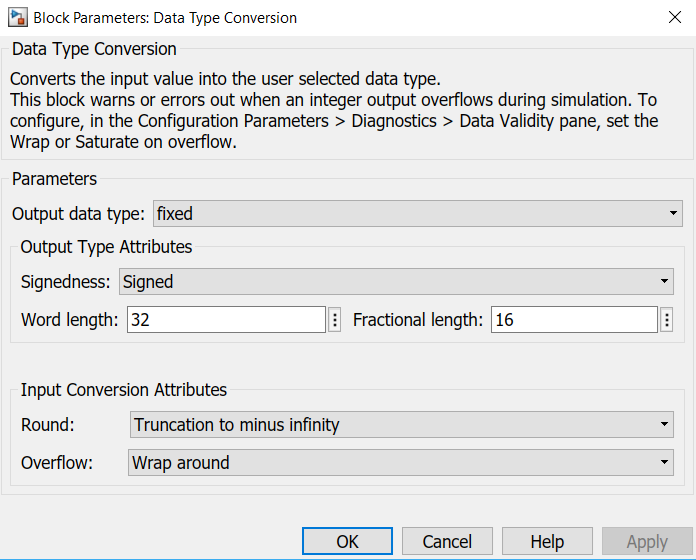4. Click OK and press Ctrl+D. Observe the signal dimensions in the design.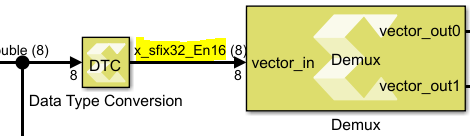To make sure the output is correct, run the simulation and observe that the same block can still be used in a generic way for different values of Word length and Fractional length. This is possible only because we have templatized the `W` and `I` values in our `C` design.

15. For an additional understanding of template parameters, perform the following:
1. Right-click the canvas and select Model Configuration Parameters to open the Model Properties window.
2. In the Model Properties window, click the Callbacks tab and select initFcn and edit the SSR value from 8 to 16 as shown in the following figure.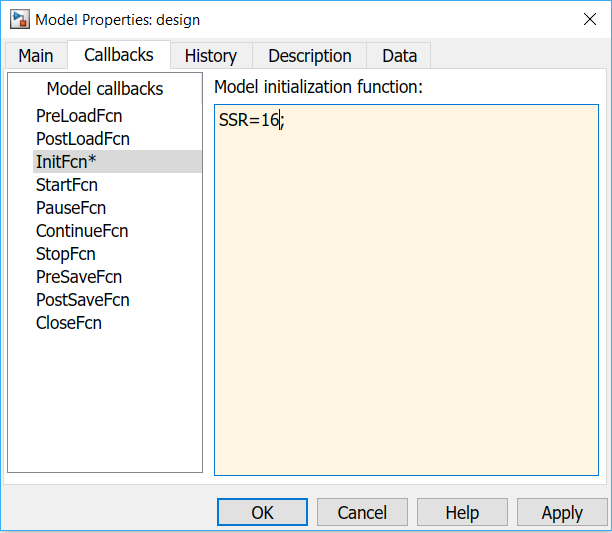3. Click OK and press Ctrl+D to observe the change in the number of elements in the Constant block output vector. The bitwidth changes when we change the datatype on the input DTC. This is possible only because of the template parameter `NUMOFELEMENTS`.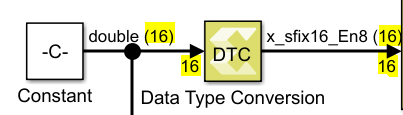4. Run the simulation and validate the output according to the input values.
Note: For information about features such as function templates for data types and pragmas to specify which data type a template variable supports, see Vitis Model Composer User Guide (UG1483).